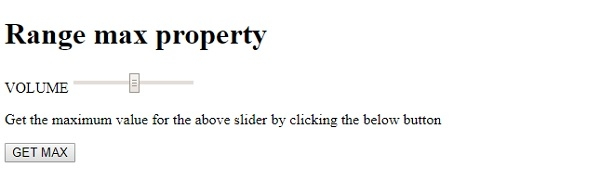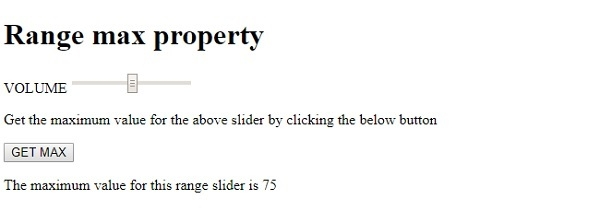# HTML DOM Input Range max property

HTMLWeb DevelopmentFront End Technology

The HTML DOM Input Range max property is used for setting or returning the max attribute value for the range slider control. This attribute is used for indicating the maximum value of the slider control and is often used with min property to create a range of values between which the slider can move.

## Syntax

Following is the syntax for −

Setting the Range max property −

rangeObject.max = number

Here, number represents maximum slider control value.

## Example

Let us look at an example for the Input range max property −

Live Demo

<!DOCTYPE html>
<html>
<body>
<h1>Range max property</h1>
<form>
VOLUME <input type="range" id="RANGE1" name="VOL" max="75">
</form>
<p>Get the maximum value for the above slider by clicking the below button.</p>
<button type="button" onclick="maxVal()">GET MAX</button>
<p id="Sample"> </p>
<script>
function maxVal() {
var R= document.getElementById("RANGE1").max;
document.getElementById("Sample").innerHTML = "The maximum value for this range slider is "+R;
}
</script>
</body>
</html>

## Output

This will produce the following output −On clicking the GET MAX button −In the above example −

We have created an input field contained inside a form having type=“range”, id=“RANGE1” , name=“VOL” and max attribute value set to 75 −

<form>
VOLUME <input type="range" id="RANGE1" name="VOL" max="75">
<form>

We have then created a button GET MAX that will execute the maxVal() method when clicked by the user −

<button type="button" onclick="maxVal()">GET MAX</button>

The maxVal() method uses the getElementById() method to get the input field with type range and its max attribute value. The returned value which signifies the maximum value the slider can have is then assigned to variable R. This value is then displayed in the paragraph with id “Sample” using its innerHTML property −

function maxVal() {
var R= document.getElementById("RANGE1").max;
document.getElementById("Sample").innerHTML = "The maximum value for this range slider is "+R;
}